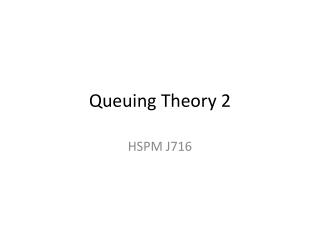DownloadDownload PresentationQueuing Theory 2

# Queuing Theory 2

Download Presentation## Queuing Theory 2

- - - - - - - - - - - - - - - - - - - - - - - - - - - E N D - - - - - - - - - - - - - - - - - - - - - - - - - - -
##### Presentation Transcript

1. Queuing Theory 2 HSPM J716

2. Customers in System and in Queue • L – mean customers in system = • Lq – mean customers in queue = L-ρ (not L-1) • There are usually fewer in the system than L, and fewer in line than Lq ,because the probability of n in system is skewed.

3. Expand from basic model • More than one server • in parallel (one queue to many servers) • in series (queues in series or stages) • Limited number in system • Limited customer population • Constant service time • Priority classes, rather than simple FIFO • (Arrivals in clumps -- not this year)

4. Multiple parallel servers

5. M servers • ρ = λ/(Mμ) ρ is how busy each server is • Probability of 0 in system:

6. 2 servers • ρ = λ/(2μ) ρ is how busy each server is • Probability of 0 in system:

7. M servers • Probability of n in the system • If n ≤ M (P(0))(Mρ)n/n! • If n ≥ M (P(0))MMρn/M!

8. 2 servers • Probability of n in the system • If n = 1 (P(0))2ρ • If n ≥ 2 (P(0))4ρn/2

9. M servers • Lq = • L = Lq + λ/μ • Wq = Lq/λ • W = Wq + 1/μ

10. 2 servers • Lq = • L = Lq + λ/μ • Wq = Lq/λ • W = Wq + 1/μ

11. Examples • a 2nd pharmacist • Burger King vs. McDonald’s: • 1 line to 2 servers vs. 2 lines to 2 servers. • 2 slow servers vs. 1 server who is twice as fast • How many seats in the cafeteria? • E.g. 1 customer per minute, 15 min. to eat, 15 seats? • How they save when you eat faster • Comfortable chairs?

12. Cookbook • Pdf version – cell references • Named cells version

13. Cookbook contents • One server (like assignment 7A) • One server, arrivals from limited group • One server, limited queue length (“balking”) • One server, constant service time • Stages of service, queue only at start • Parallel servers, one queue (Post Office) • Parallel servers, no queue (hotel) • Priority classes for arrivals